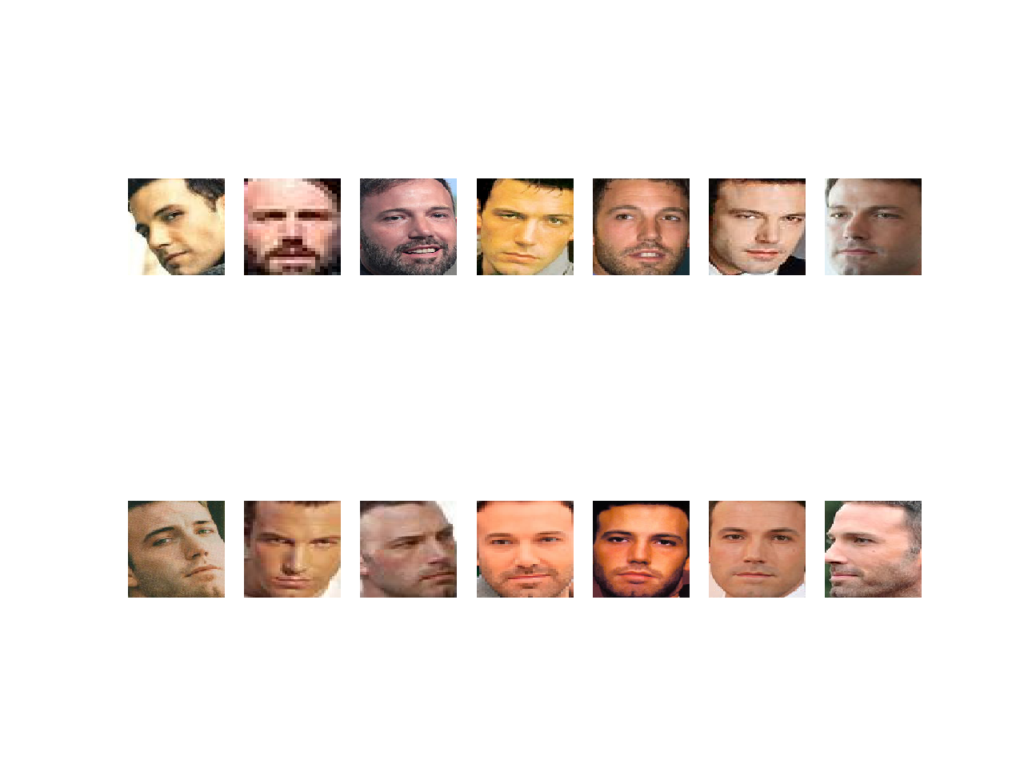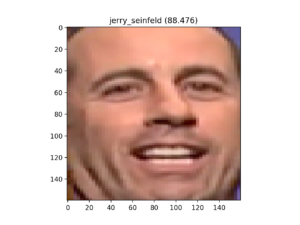# 如何在 Keras 中使用 FaceNet 开发人脸识别系统Keras 是一个用 Python 编写的高级神经网络 API，能够以 TensorFlow、CNTK 或 Theano 作为后端运行。FaceNet 是 Google 工程师 Florian Schroff、Dmitry Kalenichenko、James Philbin 等人于 2015 年开发的人脸识别系统，由于算法原理容易理解、应用方便，成了目前最为流行的人脸识别技术。今天，AI 前线带领大家跟随 Jason Brownlee 去学习如何在 Keras 中使用 FaceNet 开发人脸识别系统。

FaceNet 是 Google 研究人员于 2015 年开发的人脸识别系统，这个系统在一系列人脸识别基准数据集上取得了当时最好的成绩。由于该模型的多个第三方开源实现和预训练模型的可用性，FaceNet 系统得到了广泛的使用。

FaceNet 系统可从人脸中提取高质量的特征，称为人脸嵌入（face embeddings），可用于训练人脸识别系统。

## 教程概述

1. 人脸识别

2. FaceNet 模型

3. 如何在 Keras 中加载 FaceNet 模型

4. 如何检测人脸进行人脸识别

5. 如何开发人脸分类系统

## 人脸识别

2011 年出版的一本书 “ Handbook of Face Recognition ”（《人脸识别手册》）描述了人脸识别的两种主要模式：

• 人脸验证：给定人脸与已知身份的一对一映射（例如，这幅人脸是此人吗？）

• 人脸识别：给定人脸与已知人脸数据库的一对多映射（例如，此人是谁？）

（1）人脸验证（或身份验证）；

（2）人脸识别（或身份识别）。

——第一页， Handbook of Face Recognition ，2011 年。

## FaceNet 模型

FaceNet 是 Florian Schroff 等人在 Google 于 2015 年发表的论文 “ FaceNet: A Unified Embedding for Face Recognition and Clustering ”（《FaceNet：人脸识别与聚类的统一嵌入》）中描述的一种人脸识别系统。

FaceNet，它可以直接学习从人脸图像到紧凑的欧几里得空间的映射，其中距离直接对应于人脸相似性的度量。

## 如何在 Keras 中加载 FaceNet 模型

Keras FaceNet 预训练模型 (88 MB) 下载网址如下：

# example of loading the keras facenet modelfrom keras.models import load_model# load the modelmodel  =  load_model('facenet_keras.h5')# summarize input and output shapeprint(model.inputs)print(model.outputs)

# [<tf.Tensor 'input_1:0' shape=(?, 160, 160, 3) dtype=float32>]# [<tf.Tensor 'Bottleneck_BatchNorm/cond/Merge:0' shape=(?, 128) dtype=float32>]

## 人脸识别中如何检测人脸

sudo pip install mtcnn

# confirm mtcnn was installed correctlyimport mtcnn# print versionprint(mtcnn.__version__)

0.0.8

# load image from fileimage  =  Image.open(filename)# convert to RGB, if neededimage  =  image.convert('RGB')# convert to arraypixels  =  asarray(image)

# create the detector, using default weightsdetector  =  MTCNN()# detect faces in the imageresults  =  detector.detect_faces(pixels)

# extract the bounding box from the first facex1,  y1,  width,  height  =  results 0 # bug fixx1,  y1  =  abs(x1),  abs(y1)x2,  y2  =  x1  +  width,  y1  +  height

# extract the faceface  =  pixels[y1:y2,  x1:x2]

# resize pixels to the model sizeimage  =  Image.fromarray(face)image  =  image.resize((160,  160))face_array  =  asarray(image)

# function for face detection with mtcnnfrom PIL import Imagefrom numpy import asarrayfrom mtcnn.mtcnn import MTCNN # extract a single face from a given photographdef extract_face(filename,  required_size=(160,  160)):    # load image from file    image  =  Image.open(filename)    # convert to RGB, if needed    image  =  image.convert('RGB')    # convert to array    pixels  =  asarray(image)    # create the detector, using default weights    detector  =  MTCNN()    # detect faces in the image    results  =  detector.detect_faces(pixels)    # extract the bounding box from the first face    x1,  y1,  width,  height  =  results 0     # bug fix    x1,  y1  =  abs(x1),  abs(y1)    x2,  y2  =  x1  +  width,  y1  +  height    # extract the face    face  =  pixels[y1:y2,  x1:x2]    # resize pixels to the model size    image  =  Image.fromarray(face)    image  =  image.resize(required_size)    face_array  =  asarray(image)    return  face_array # load the photo and extract the facepixels  =  extract_face('...')

## 如何开发人脸分类系统

### 5 Celebrity Faces Dataset

The 5 Celebrity Faces Dataset 是一个包含名人照片的小型数据集。

5-celebrity-faces-dataset├── train│   ├── ben_afflek│   ├── elton_john│   ├── jerry_seinfeld│   ├── madonna│   └── mindy_kaling└── val    ├── ben_afflek    ├── elton_john    ├── jerry_seinfeld    ├── madonna    └── mindy_kaling

### 检测人脸

# demonstrate face detection on 5 Celebrity Faces Datasetfrom os import listdirfrom PIL import Imagefrom numpy import asarrayfrom matplotlib import pyplotfrom mtcnn.mtcnn import MTCNN # extract a single face from a given photographdef extract_face(filename,  required_size=(160,  160)):    # load image from file    image  =  Image.open(filename)    # convert to RGB, if needed    image  =  image.convert('RGB')    # convert to array    pixels  =  asarray(image)    # create the detector, using default weights    detector  =  MTCNN()    # detect faces in the image    results  =  detector.detect_faces(pixels)    # extract the bounding box from the first face    x1,  y1,  width,  height  =  results 0     # bug fix    x1,  y1  =  abs(x1),  abs(y1)    x2,  y2  =  x1  +  width,  y1  +  height    # extract the face    face  =  pixels[y1:y2,  x1:x2]    # resize pixels to the model size    image  =  Image.fromarray(face)    image  =  image.resize(required_size)    face_array  =  asarray(image)    return  face_array # specify folder to plotfolder  =  '5-celebrity-faces-dataset/train/ben_afflek/'i  =  1# enumerate filesfor  filename in  listdir(folder):    # path    path  =  folder  +  filename    # get face    face  =  extract_face(path)    print(i,  face.shape)    # plot    pyplot.subplot(2,  7,  i)    pyplot.axis('off')    pyplot.imshow(face)    i  +=  1pyplot.show()

1 (160, 160, 3)2 (160, 160, 3)3 (160, 160, 3)4 (160, 160, 3)5 (160, 160, 3)6 (160, 160, 3)7 (160, 160, 3)8 (160, 160, 3)9 (160, 160, 3)10 (160, 160, 3)11 (160, 160, 3)12 (160, 160, 3)13 (160, 160, 3)14 (160, 160, 3)# load images and extract faces for all images in a directorydef load_faces(directory):    faces  =  list()    # enumerate files    for  filename in  listdir(directory):        # path        path  =  directory  +  filename        # get face        face  =  extract_face(path)        # store        faces.append(face)    return  faces

# load a dataset that contains one subdir for each class that in turn contains imagesdef load_dataset(directory):    X,  y  =  list(),  list()    # enumerate folders, on per class    for  subdir in  listdir(directory):        # path        path  =  directory  +  subdir  +  '/'        # skip any files that might be in the dir        if  not  isdir(path):            continue        # load all faces in the subdirectory        faces  =  load_faces(path)        # create labels        labels  =  [subdir for  _  in  range(len(faces))]        # summarize progress        print('>loaded %d examples for class: %s'  %  (len(faces),  subdir))        # store        X.extend(faces)        y.extend(labels)    return  asarray(X),  asarray(y)

# load train datasettrainX,  trainy  =  load_dataset('5-celebrity-faces-dataset/train/')print(trainX.shape,  trainy.shape)# load test datasettestX,  testy  =  load_dataset('5-celebrity-faces-dataset/val/')print(testX.shape,  testy.shape)# save arrays to one file in compressed formatsavez_compressed('5-celebrity-faces-dataset.npz',  trainX,  trainy,  testX,  testy)

# face detection for the 5 Celebrity Faces Datasetfrom os import listdirfrom os.path import isdirfrom PIL import Imagefrom matplotlib import pyplotfrom numpy import savez_compressedfrom numpy import asarrayfrom mtcnn.mtcnn import MTCNN # extract a single face from a given photographdef extract_face(filename,  required_size=(160,  160)):    # load image from file    image  =  Image.open(filename)    # convert to RGB, if needed    image  =  image.convert('RGB')    # convert to array    pixels  =  asarray(image)    # create the detector, using default weights    detector  =  MTCNN()    # detect faces in the image    results  =  detector.detect_faces(pixels)    # extract the bounding box from the first face    x1,  y1,  width,  height  =  results 0     # bug fix    x1,  y1  =  abs(x1),  abs(y1)    x2,  y2  =  x1  +  width,  y1  +  height    # extract the face    face  =  pixels[y1:y2,  x1:x2]    # resize pixels to the model size    image  =  Image.fromarray(face)    image  =  image.resize(required_size)    face_array  =  asarray(image)    return  face_array # load images and extract faces for all images in a directorydef load_faces(directory):    faces  =  list()    # enumerate files    for  filename in  listdir(directory):        # path        path  =  directory  +  filename        # get face        face  =  extract_face(path)        # store        faces.append(face)    return  faces # load a dataset that contains one subdir for each class that in turn contains imagesdef load_dataset(directory):    X,  y  =  list(),  list()    # enumerate folders, on per class    for  subdir in  listdir(directory):        # path        path  =  directory  +  subdir  +  '/'        # skip any files that might be in the dir        if  not  isdir(path):            continue        # load all faces in the subdirectory        faces  =  load_faces(path)        # create labels        labels  =  [subdir for  _  in  range(len(faces))]        # summarize progress        print('>loaded %d examples for class: %s'  %  (len(faces),  subdir))        # store        X.extend(faces)        y.extend(labels)    return  asarray(X),  asarray(y) # load train datasettrainX,  trainy  =  load_dataset('5-celebrity-faces-dataset/train/')print(trainX.shape,  trainy.shape)# load test datasettestX,  testy  =  load_dataset('5-celebrity-faces-dataset/val/')# save arrays to one file in compressed formatsavez_compressed('5-celebrity-faces-dataset.npz',  trainX,  trainy,  testX,  testy)

>loaded 14 examples for class: ben_afflek>loaded 19 examples for class: madonna>loaded 17 examples for class: elton_john>loaded 22 examples for class: mindy_kaling>loaded 21 examples for class: jerry_seinfeld(93, 160, 160, 3) (93,)>loaded 5 examples for class: ben_afflek>loaded 5 examples for class: madonna>loaded 5 examples for class: elton_john>loaded 5 examples for class: mindy_kaling>loaded 5 examples for class: jerry_seinfeld(25, 160, 160, 3) (25,)

### 创建人脸嵌入

FaceNet 模型可以用作分类其本身的一部分，或者，我们可以用 FaceNet 模型对人脸进行预处理，创建可以存储并用作分类器模型输入的人脸嵌入。首选后一种方法，因为 FaceNet 模型既大又慢，不利于创建人脸嵌入。

# load the face datasetdata  =  load('5-celebrity-faces-dataset.npz')trainX,  trainy,  testX,  testy  =  data['arr_0'],  data['arr_1'],  data['arr_2'],  data['arr_3']print('Loaded: ',  trainX.shape,  trainy.shape,  testX.shape,  testy.shape)

# load the facenet modelmodel  =  load_model('facenet_keras.h5')print('Loaded Model')

# scale pixel valuesface_pixels  =  face_pixels.astype('float32')# standardize pixel values across channels (global)mean,  std  =  face_pixels.mean(),  face_pixels.std()face_pixels  =  (face_pixels  -  mean)  /  std

# transform face into one samplesamples  =  expand_dims(face_pixels,  axis=0)

# make prediction to get embeddingyhat  =  model.predict(samples)# get embeddingembedding  =  yhat

# get the face embedding for one facedef get_embedding(model,  face_pixels):    # scale pixel values    face_pixels  =  face_pixels.astype('float32')    # standardize pixel values across channels (global)    mean,  std  =  face_pixels.mean(),  face_pixels.std()    face_pixels  =  (face_pixels  -  mean)  /  std    # transform face into one sample    samples  =  expand_dims(face_pixels,  axis=0)    # make prediction to get embedding    yhat  =  model.predict(samples)    return  yhat

# calculate a face embedding for each face in the dataset using facenetfrom numpy import loadfrom numpy import expand_dimsfrom numpy import asarrayfrom numpy import savez_compressedfrom keras.models import load_model # get the face embedding for one facedef get_embedding(model,  face_pixels):    # scale pixel values    face_pixels  =  face_pixels.astype('float32')    # standardize pixel values across channels (global)    mean,  std  =  face_pixels.mean(),  face_pixels.std()    face_pixels  =  (face_pixels  -  mean)  /  std    # transform face into one sample    samples  =  expand_dims(face_pixels,  axis=0)    # make prediction to get embedding    yhat  =  model.predict(samples)    return  yhat # load the face datasetdata  =  load('5-celebrity-faces-dataset.npz')trainX,  trainy,  testX,  testy  =  data['arr_0'],  data['arr_1'],  data['arr_2'],  data['arr_3']print('Loaded: ',  trainX.shape,  trainy.shape,  testX.shape,  testy.shape)# load the facenet modelmodel  =  load_model('facenet_keras.h5')print('Loaded Model')# convert each face in the train set to an embeddingnewTrainX  =  list()for  face_pixels in  trainX:    embedding  =  get_embedding(model,  face_pixels)    newTrainX.append(embedding)newTrainX  =  asarray(newTrainX)print(newTrainX.shape)# convert each face in the test set to an embeddingnewTestX  =  list()for  face_pixels in  testX:    embedding  =  get_embedding(model,  face_pixels)    newTestX.append(embedding)newTestX  =  asarray(newTestX)print(newTestX.shape)# save arrays to one file in compressed formatsavez_compressed('5-celebrity-faces-embeddings.npz',  newTrainX,  trainy,  newTestX,  testy)

Loaded:  (93, 160, 160, 3) (93,) (25, 160, 160, 3) (25,)Loaded Model(93, 128)(25, 128)

### 执行人脸分类

# load datasetdata  =  load('5-celebrity-faces-embeddings.npz')trainX,  trainy,  testX,  testy  =  data['arr_0'],  data['arr_1'],  data['arr_2'],  data['arr_3']print('Dataset: train=%d, test=%d'  %  (trainX.shape,  testX.shape))

# normalize input vectorsin_encoder  =  Normalizer(norm='l2')trainX  =  in_encoder.transform(trainX)testX  =  in_encoder.transform(testX)

# label encode targetsout_encoder  =  LabelEncoder()out_encoder.fit(trainy)trainy  =  out_encoder.transform(trainy)testy  =  out_encoder.transform(testy)

# fit modelmodel  =  SVC(kernel='linear')model.fit(trainX,  trainy)

# predictyhat_train  =  model.predict(trainX)yhat_test  =  model.predict(testX)# scorescore_train  =  accuracy_score(trainy,  yhat_train)score_test  =  accuracy_score(testy,  yhat_test)# summarizeprint('Accuracy: train=%.3f, test=%.3f'  %  (score_train*100,  score_test*100))

# develop a classifier for the 5 Celebrity Faces Datasetfrom numpy import loadfrom sklearn.metrics import accuracy_scorefrom sklearn.preprocessing import LabelEncoderfrom sklearn.preprocessing import Normalizerfrom sklearn.svm import SVC# load datasetdata  =  load('5-celebrity-faces-embeddings.npz')trainX,  trainy,  testX,  testy  =  data['arr_0'],  data['arr_1'],  data['arr_2'],  data['arr_3']print('Dataset: train=%d, test=%d'  %  (trainX.shape,  testX.shape))# normalize input vectorsin_encoder  =  Normalizer(norm='l2')trainX  =  in_encoder.transform(trainX)testX  =  in_encoder.transform(testX)# label encode targetsout_encoder  =  LabelEncoder()out_encoder.fit(trainy)trainy  =  out_encoder.transform(trainy)testy  =  out_encoder.transform(testy)# fit modelmodel  =  SVC(kernel='linear',  probability=True)model.fit(trainX,  trainy)# predictyhat_train  =  model.predict(trainX)yhat_test  =  model.predict(testX)# scorescore_train  =  accuracy_score(trainy,  yhat_train)score_test  =  accuracy_score(testy,  yhat_test)# summarizeprint('Accuracy: train=%.3f, test=%.3f'  %  (score_train*100,  score_test*100))

Dataset: train=93, test=25Accuracy: train=100.000, test=100.000

# load facesdata  =  load('5-celebrity-faces-dataset.npz')testX_faces  =  data['arr_2']

# test model on a random example from the test datasetselection  =  choice([i  for  i  in  range(testX.shape)])random_face_pixels  =  testX_faces[selection]random_face_emb  =  testX[selection]random_face_class  =  testy[selection]random_face_name  =  out_encoder.inverse_transform([random_face_class])

# prediction for the facesamples  =  expand_dims(random_face_emb,  axis=0)yhat_class  =  model.predict(samples)yhat_prob  =  model.predict_proba(samples)

# get nameclass_index  =  yhat_classclass_probability  =  yhat_prob[0,class_index]  *  100predict_names  =  out_encoder.inverse_transform(yhat_class)

print('Predicted: %s (%.3f)'  %  (predict_names,  class_probability))print('Expected: %s'  %  random_face_name)

# plot for funpyplot.imshow(random_face_pixels)title  =  '%s (%.3f)'  %  (predict_names,  class_probability)pyplot.title(title)pyplot.show()

# develop a classifier for the 5 Celebrity Faces Datasetfrom random import choicefrom numpy import loadfrom numpy import expand_dimsfrom sklearn.preprocessing import LabelEncoderfrom sklearn.preprocessing import Normalizerfrom sklearn.svm import SVCfrom matplotlib import pyplot# load facesdata  =  load('5-celebrity-faces-dataset.npz')testX_faces  =  data['arr_2']# load face embeddingsdata  =  load('5-celebrity-faces-embeddings.npz')trainX,  trainy,  testX,  testy  =  data['arr_0'],  data['arr_1'],  data['arr_2'],  data['arr_3']# normalize input vectorsin_encoder  =  Normalizer(norm='l2')trainX  =  in_encoder.transform(trainX)testX  =  in_encoder.transform(testX)# label encode targetsout_encoder  =  LabelEncoder()out_encoder.fit(trainy)trainy  =  out_encoder.transform(trainy)testy  =  out_encoder.transform(testy)# fit modelmodel  =  SVC(kernel='linear',  probability=True)model.fit(trainX,  trainy)# test model on a random example from the test datasetselection  =  choice([i  for  i  in  range(testX.shape)])random_face_pixels  =  testX_faces[selection]random_face_emb  =  testX[selection]random_face_class  =  testy[selection]random_face_name  =  out_encoder.inverse_transform([random_face_class])# prediction for the facesamples  =  expand_dims(random_face_emb,  axis=0)yhat_class  =  model.predict(samples)yhat_prob  =  model.predict_proba(samples)# get nameclass_index  =  yhat_classclass_probability  =  yhat_prob[0,class_index]  *  100predict_names  =  out_encoder.inverse_transform(yhat_class)print('Predicted: %s (%.3f)'  %  (predict_names,  class_probability))print('Expected: %s'  %  random_face_name)# plot for funpyplot.imshow(random_face_pixels)title  =  '%s (%.3f)'  %  (predict_names,  class_probability)pyplot.title(title)pyplot.show()

Predicted: jerry_seinfeld (88.476)Expected: jerry_seinfeld## 延伸阅读

### API

Jason Brownlee 博士，机器学习专家，他通过实践教程教授开发人员如何使用现代机器学习方法来获得结果。